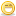A Flash Developer Resource Site

# Thread: Trying to learn a new Chemistry concept

1. ##Just started to do these types of problems in Chem class. I know this is a math and physics forum, but I hope maybe someone could help me.

4.1 mL of 0.54 molar H2SO4 (sulfuric acid) are required to neutralize 5.0 mL of NaOH. What is the molarity of the NaOH?

I would greatly appreciate any explanation.

ThanksReply With Quote

2. its been a while since chemistry, but let me see if i can remember.

molarity = moles / volume, so you can find the number of moles of by taking molarity times volume. in order for the acid to neutralize the base, the moles have to equal each other. so i believe that you can just set up a little equation like:
V1M1=V2M2 where v is the volume IN LITERS and m is the molarity
in your case, the equation would be:
(.0041)(0.54)=(.0050)x or:
x = ((.0042)(0.54))/(.0050)
i get .4536

if this is not right, sorry. it has been awhileReply With Quote

3. minger - almost....

the fact is that we need to know the equation for the reaction....

H2SO4 + 2NaOH = Na2SO4 + 2H20 (though the equals should be an arrow)

so 1 mole of acid reacts with 2 moles of alkali:

remembering this, number of moles of acid = 0.54 * 4.1 (*10e-3) = 2.214 (*10e-3)moles

Therefore number of moles of alkali must equal 2*2.214(*10e-3)= 4.428 (*10e-3)

and as molarity = moles/volume,

molarity of alkali = 4.428(*10e-3)/5(*10e-3)

= 0.886 Molar

Hard to write the process correctly here without the subs and supers, and I've put the standard form for the conversion of millilitres into litres, which you should do, though you'll notice they cancel in the final sum.

So - REMEMBER - write the correct equation (balanced of course) down first.

swillsReply With Quote

####Posting Permissions

• You may not post new threads
• You may not post replies
• You may not post attachments
• You may not edit your posts
•

 » Home » Movies » Tutorials » Submissions » Board » Links » Reviews » Feedback » Gallery » Fonts » The Lounge » Sound Loops » Sound FX » About FK » Sitemap